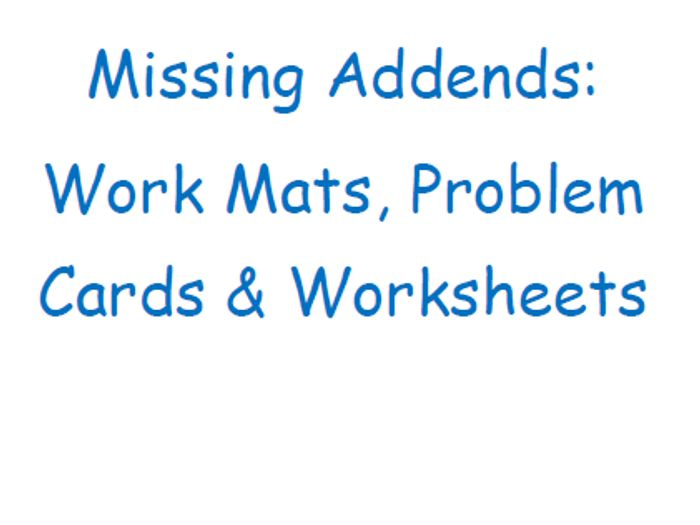This set is to provide students with additional practice determining unknown addends in problems with 2 and 3 addends.
There are 3 different Work Mats students use to work out problems with missing addends. They are for: problems with 2 addends, problems with 3 addends and vertical problems with 2 addends.
There are 108 pages of Missing Addend problems. For my class, I print out one copy and divide the problems among my groups since there are so many problems. There are 6-8 problems per page. (about 620 problems). The problems have sums from 5 to 20 and cover 2 addend problems and 3 addend problems, as well as, 2 addend vertical problems.
There are also 3 worksheets for students to do.
Thanks!
\$5.00
Save for later

### Info

Created: May 3, 2017

Updated: Feb 22, 2018

pdf, 371 KB